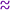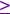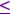AW starts with the designation of goods or issues in a dispute. The parties then indicate how much they value obtaining the different goods, or "getting their way" on the different issues, by distributing 100 points across them. This information, which may or may not be made public, becomes the basis for fairly dividing the goods and issues later. Once the points have been assigned by both parties (in secret), a mediator (or a computer) can use AW to allocate the goods to each party, and to determine which good (there will be at most one) that may need to be divided.

Let's illustrate the procedure with an example. Suppose Bob and Carol are getting a divorce and must divide up some of their assets. We assume that they distribute 100 points among the five items as follows:

 Item Carol Bob Retirement Account 50 40 Home 20 30 Summer Cottage 15 10 Investments 10 10 Other 5 10 Total 100 100

AW works by assigning, initially, the item to the person who puts more points on it (that person's points are underlined above). Thus, Bob gets the home, because he placed 30 points on it compared to Carol's 20. Likewise, Bob also gets the items in the "other" category, whereas Carol gets the retirement account and the summer cottage. Leaving aside the tied item (investments), Carol has a total of 65 (50 + 15) of her points, and Bob a total of 40 (30 + 10) of his points. This completes the "winner" phase of adjusted winner.

Because Bob trails Carol in points (40 compared to 65) in this phase, initially we award the investments on which they tie to Bob, which brings him up to 50 points (30 + 10 + 10). Now we will start the "adjusted" phase of AW. The goal of this phase is to achieve an equitable allocation by transferring items, or fractions thereof, from Carol to Bob until their points are equal.

What is important here is the order in which items are transferred. This order is determined by looking at certain fractions, corresponding to the items that Carol, the initial winner, has and may have to give up. In particular, for each item Carol won initially, we look at the fraction giving the ratio of Carol's points to Bob's for that item:

(Number of points Carol assigned to the item)/(Number of points Bob assigned to the item)

In our example, Carol won two items, the retirement account and the summer cottage. For the retirement account, the fraction is 50/40 = 1.25, and for the summer cottage the fraction is 15/10 = 1.50.

We start by transferring items from Carol to Bob, beginning with the item with the smallest fraction. This is the retirement account, with a fraction equal to 1.25. We continue transferring goods until the point totals are equal.

Notice that if we transferred the entire retirement account from Carol to Bob, Bob would wind up with 90 (50 + 40) of his points, whereas Carol would plunge to 15 (65 - 50) of her points. We conclude, therefore, that the parties will have to share or split the item. So our task is to find exactly what fraction of this item each party will get so that their point totals come out to be equal.

We can use algebra to find the solution. Let p be the fraction (or percentage) of the retirement account that we need to transfer from Carol to Bob in order to equalize totals; in other words, p is the fraction of the retirement account that Bob will get, and (1-p) is the fraction that Carol will get. After the transfer, Bob's point total will be 50 + 40p, and Carol's point total will be 15 + 50(1-p). Since we want the point totals to be equal, we want to choose p so that it satisfies

50 + 40p = 15 + 50(1-p)

Solving for p we get

90p = 15

p = 15/90 = 1/6

Thus, Bob should get 1/6 of the retirement account and Carol should get the remaining 5/6.

Recall that initially Bob is receiving: (1) the home (30 points), (2) the "other" items (10 points), and (3) the investments (10 points). Together with 1/6 of the retirement account, Bob's point total is now

30 + 10 + 10 + 40(1/6) = 50 + 40(1/6)50 + 6.67 = 56.67

Recall that initially Carol is receiving: (1) the summer cottage (15 points). Together with 5/6 of the retirement account, Carol's point total is now

15 + 50(5/6)15 + 41.67 = 56.67

Thus, each person receives exactly the same number of points, as he or she values their allocations.

Suppose that Bob and Carol want to fairly divide k goods, where k2. We will illustrate the general description below with additional examples.

AW allocates goods as follows:

1. Each player is given 100 points to assign to each good as he/she sees fit.

2. Suppose X is sum of the points of all goods that Bob reports that he values more than Carol does. Let Y be the sum of the values of the goods that Carol reports that she values more than Bob does. Assume XY. Assign the goods so that Bob initially gets all the goods where xiyi, and Carol gets the others.

3. List the goods in an order G1,G2,..., so that the following holds:

• Bob, based on his reported values, values goods G1,...,Gr at least as much as Carol does (i.e. xiyi forir)

• Carol, based on her reported values, values goods Gr+1,...,Gk at least as much as Bob does (i.e. yixi for r+1ik)

• x1/yx2/y. . .xr/yr

4. Transfer as much of G1 from Bob to Carol as needed to achieve equitability -- that is , until the point totals of the two players are equal. If the scores are not equal after transferring all of G1 to Carol, transfer as much of G2, G3, etc., as needed.

The AW procedure satisfies the following properties:

• Envy-free: Bob and Carol both believe that their portion is at least tied for largest (based on their announced valuations)
• Equitability: Bob and Carol both believe that their portion is valued the same as the other player's (based on their announced valuations)
• Efficient: There is no allocation that is strictly better for Bob (or Carol) and as good for Carol (or Bob)

Examples

Suppose Bob and Carol are dividing three goods: A, B, and C.

1. They report the following point assignments:

 Item Bob's reported values Carol's reported value A 6 5 B 67 34 C 27 61 Total 100 100

2. X = 6+67 = 73 and Y = 61 so, initially Bob is assigned A and B, giving him 73 points, and Carol is assigned item C, giving her 61 points.
3. Since 6/5 = 1.2 and 67/34 = 1.97, order the goods: (1) A, (2) B, and (3) C.
4. We first transfer item A from Bob to Carol, so Bob has 67 points and Carol has 66 points (61 + 5). This allocation is not equitable, so we need to transfer part of item B from Bob to Carol. Let p denote the proportion of B that Bob will keep, and (1-p) the proportion that Carol will keep. Then Bob's point total will be 67p, and Carol's point total will be 61 + 5 + 34(1-p) = 100 - 34p. We will choose p so that

67p = 100 - 34p

yielding p = 100/101, which is approximately equal to 0.99.

Thus, Bob ends up with 99% of item B for a total of 66.3 of his points (67 x 0.99), whereas Carol ends up with all of item C, all of item A, and 1% of item B for a total of 66.3 of her points [61 + 5 + ( 34 x 0.01)].

Check the properties

• Envy-free: Bob would not trade his allocation for Carol's allocation, since that would give him only 33 points. Similarly, Carol would not trade her allocation for Bob's allocation, since that would give her only 34 points.

• Equitable: This property is obviously satisfied since both parties receive 66.3 points.

• Efficient: This is more difficult to show, because it requires showing that there can be no better allocation for both players. The formal proof can be found in the book Fair Division by Brams and Taylor. It should be noted that the initial allocation is efficient, since each player receives all the goods he or she most values, and the equitability adjustment step does not affect efficiency.